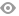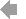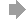Basic HTML VersionView Full Version
Page 9 - American Punch Ironworker Tooling Catalog
P. 9

``````PUNCHING TIPS
HOW TO TO CALCULATE REQUIRED TONNAGE FOR PUNCHING MILDSTEELWITHTENSILESTRENGTHOF65 000PSI
Z = L - W ROUND:
Hole Size X X Material Thickness X X 102 = Tonnage OBLONG:
W L [( 63 X X X Z) + “W”] X X X Material Thickness X X X 102 = Tonnage SQUARE:
For For tensile tensile strength strength of of 85 000 000 exchange exchange 102 102 with with 134 A A X X X 1 1 27 X X X Material Thickness X X X 102 =Tonnage RECTANGLE:
(L + W) X X X 64 X X X Material Thickness X X X 102 = Tonnage For For tensile tensile strength strength of of 75 000 000 exchange exchange 102 102 with with 118 A HOW TO CALCULATE THE DIAGONAL DIMENSION OF A A A A A PUNCH The diagonal dimension of a a a a square rectangular or hex punch can not exceed the the punch body or or bore of the the coupling nut Here’s how to determine the diagonal dimension of a a a punch:
SQUARE Diagonal
Size Size X 1 1 414 = Diagonal
RECTANGULAR
Diagonal
Size L2 + W 2= Diagonal
HEXAGONAL
Diagonal
Size X 1 1 154 = Diagonal
Size HOW TO CALCULATE THE LARGEST SQUARE SIZE ALLOWED FOR A A A A A PUNCH STYLE
PUNCH STYLE
NO MAX SQUARE SIZE 116 729 119 751 121 861 128 1 1 1 104 164 884 192 662
Body
PUNCH BODY DIVIDED BY 1 1 414 = MAXIMUM SQUARE SIZE Cannot be larger than punch body Size 9 Size

``````7   8   9   10   11